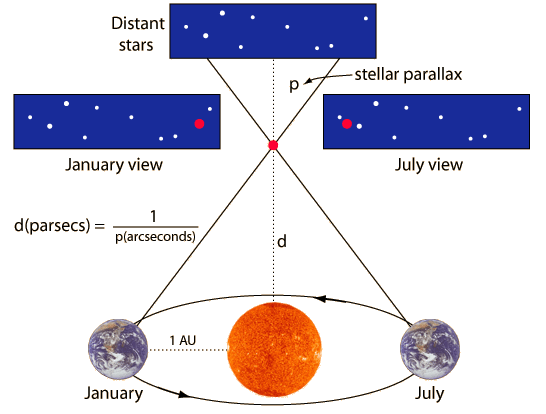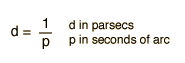# Stellar Parallax

A nearby star's apparent movement against the background of more distant stars as the Earth revolves around the Sun is referred to as stellar parallax.This exaggerated view shows how we can see the movement of nearby stars relative to the background of much more distant stars and use that movement to calculate the distance to the nearby star.

 The parallax can be used to measure the distance to the few stars which are close enough to the Sun to show a measurable parallax. The distance to the star is inversely proportional to the parallax. The distance to the star in parsecs is given byThe nearest star is proxima centauri, which exhibits a parallax of 0.762 arcsec, and therefore is 1.31 parsecs away.Some well-known examples of distance measurement by parallax are 61 Cygni at 1/3 of an arcsec, distance 3 parsecs, and Barnard's Star at 1.8 parsecs = 5.9 light years. Barnard's Star also exhibits a large proper motion.

The distance at which parallax can be reliably measured has now been greatly extended by space-based instruments like the Hipparcos satellite.

Index

Distance measurement

References
Kaufman, Universe

Chaisson & McMillan

 HyperPhysics***** Astrophysics R Nave
Go Back

# Distance Units

The average distance between the Earth and the Sun is a natural distance unit and is called an Astronomical Unit (AU).

1 AU = 1.495978 x 1011 m

The light year (ly) is another often used distant unit

1 ly = 9.46053 x 1015 m = 6.324 x 104 AU

An often preferrable large distance unit is the parsec (pc) , the distance at which one AU would subtend a second of arc:

1 pc = 3.085678 x 1016 m = 3.261633 ly = 206265 AU

 Magnitude Time Units
Index

Distance measurement

 HyperPhysics***** Astrophysics R Nave
Go Back

# Proper Motion

The change in a star's position in the sky as a result of its true motion through space is called proper motion. This is distinguished from the annual apparent motion in the sky caused by the Earth's orbit around the Sun. This apparent motion permits distance measurement by parallax.

The largest proper motion is exhibited by Barnard's Star which is about 5.9 light years from the Earth. Barnard Star's proper motion is 10.3 arcseconds per year, taking only 200 years to travel the angular diameter of the moon across the sky.

Index

 HyperPhysics***** Astrophysics R Nave
Go Back

# Time Units

The sidereal year = 365.2564 mean solar days = 365d 6h 9m 10s

The tropical year = 365.2422 mean solar days = 365d 5h 48m 46s

The tropical year is different from the sidereal year because of the precession of the Earth.

Index

 HyperPhysics***** Astrophysics R Nave
Go Back

# Magnitude

Magnitude is a historical unit of stellar brightness and is defined such that a change of 5 magnitudes represents a factor of 100 in intensity. First magnitude was supposed to be the brightness of the brightest stars, but with quantitative measurement some stars were found to be brighter than those used for the standard. The definition of magnitude above reduces to the following expression for change in magnitude:Magnitude change = implies x10^ times intensity.

As a number assigned to the visual brightness of an object, the magnitude is said to be the apparent magnitude. Star A will have the same apparent magnitude as star B if B has four times the luminosity but is twice as far away. The light power that reaches us drops off according to the inverse square law. To compare the intrinsic brightnesses of objects, their apparent magnitudes can be adjusted to that which would be observed at the same distance. A distance of 10 parsecs has been chosen as a convenient distance for comparison, and the magnitude an object would have at 10 pc is called its absolute magnitude.

The magnitude scale was devised by the second century Greek astronomer Hipparchus. He divided all stars into 6 magnitudes with magnitude 1 being the brightest star and magnitude 6 being the faintest star visible with the unaided eye.

 Magnitude Examples
Index

 HyperPhysics***** Astrophysics R Nave
Go Back

# Magnitude Examples

The magnitude is a comparative stellar brightness scale. First magnitude was supposed to be the brightness of the brightest stars, but with quantitative measurement some stars were found to be brighter than those used for the standard. About 6th magnitude represents the faintest stars which can be seen by the unaided eye. Some other examples are:

 Sun -26.8 Moon -12.6 Venus -4.4 Faintest for naked eye visibility + 6 Brightest quasar +12.8 Faintest star visible at Kitt Peak +24

Index

 HyperPhysics***** Astrophysics R Nave
Go Back